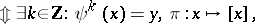Naked singularity

(diff) ← Older revision | Latest revision (diff) | Newer revision → (diff)

According to Einstein's theory of general relativity (cf. Relativity theory), space-time can be modeled by a $4$-dimensional Lorentzian manifold (cf. Pseudo-Riemannian space). Photons correspond to null geodesics and freely falling particles to time-like geodesics (cf. also Geodesic line). Since an affine parameter of a time-like geodesic can be regarded as a normal clock carried by the freely falling particle, one says that a Lorentzian manifold contains a (future) singularity if there exists a time-like (or null) geodesic $\gamma$ which is future inextensible and whose affine parameter remains finite. The interpretation of a singularity as a physical singularity in the sense that space-time or gravity diverges in some sense may be misleading. As a simple example, consider the $2$-dimensional Lorentzian manifold $( M , g ) = ( \mathbf{R} ^ { 2 } \backslash \{ 0 \} , 2 / ( u ^ { 2 } + v ^ { 2 } ) d u d v )$. The curve $\nu ( t ) : = ( 1 / ( 1 - t ) , 0 )$ is an incomplete geodesic and the mapping $\psi : ( u , v ) \rightarrow ( 2 u , 2 v )$ is an isometry (cf. also Isometric mapping). Defining $x \sim y$ as

\begin{equation*} x \sim y \end{equation*}one obtains the compact Lorentzian Clifford–Pohl torus $( \pi ( M ) , \pi_{*} g )$. The curve $\pi ( \nu )$ is an incomplete null geodesic in this compact torus and, therefore, in any physical sense a non-singular Lorentzian manifold. There are theorems which state that under "physically reasonable" conditions there are singularities in the Lorentzian manifolds representing space-times [a2], [a1] (but see also [a4] and [a3]). There is not much known about the properties of the singularities predicted by these theorems. In particular, it is not known whether these singularities are necessarily of a different nature than those in the Clifford–Pohl torus. More generally, it is not known whether they can be naked in the following sense.

A singularity is called naked if it is visible from some regular point in space-time $M$, i.e., if there is an $x \in M$ such that the geodesic $\gamma$ is contained in the past of $x$. It is presently (1998) believed by the majority of physicists that naked future singularities do not occur in physically realistic and stable space-times (cf. Penrose cosmic censorship). Note that in the physics literature there are various conflicting definitions of singularities and naked singularities. The version chosen here is motivated by the singularity theorems.

How to Cite This Entry:
Naked singularity. Encyclopedia of Mathematics. URL: http://encyclopediaofmath.org/index.php?title=Naked_singularity&oldid=50803
This article was adapted from an original article by Marcus Kriele (originator), which appeared in Encyclopedia of Mathematics - ISBN 1402006098. See original article